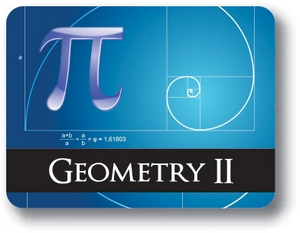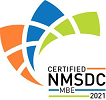Red Comet## Geometry - Semester - 2

#### Course Price: \$285.00## Course Details:

This course provides an opportunity for students to explore Trigonometry, Analytic Geometry, and Modeling. Topics include properties and solutions for right triangles, Law of Sines and Cosines and their applications; properties and construction of circles and tangents to circles, properties and construction of cyclic quadrilaterals and regular polygons; parallel and perpendicular lines, deriving their slopes and distance between them; surface area, volume, and perimeter of several types of geometric figures; describing and applying geometric measurements for practical purposes; rotating 2D objects, cross-section of 3D geometric figures; concepts of density and solving design problems using geometric concepts. Students perform constructions, measure figures, observe patterns, discuss their findings, write their own definitions, and formulate and prove geometric conjectures.

Note: This course is not designed for ELL (English Language Learners) students. ELL students may enroll in this course ONLY if they have adequate mentor support at their home school and are able to fulfill all course requirements.

## Syllabus:

Section 1: Trigonometry of triangle

Objectives:

• To use geometric mean and find lengths of segments in a right triangle
• To determine and use relations in similar right triangles
• Apply the converse of Pythagorean Theorem, to determine is a triangle is right, obtuse or acute
• To define and use six trigonometric ratios as ratios of sides of a right triangle
• To use properties of 45-45-90 degree and 30-60-90 degree triangles to solve problems
• To solve right triangles using trigonometric ratios
• To use trigonometric ratios and solve problems involving angle of elevation and angle of depression
• To find missing angles in a right triangle using inverse trigonometric ratios
• Apply the Law of Sines to solve a triangle
• Solve word problems requiring the Law of Sines
• Be able to determine the number of triangles that exist in the ambiguous case
• Apply the Law of Cosines to solve a triangle
• Solve word problems requiring the Law of Cosines

Lessons:

• Properties of Right Triangle
• Trigonometry of Right Triangles
• Special Right Triangles
• Solving Right Triangles
• Law of Sines and its Applications
• Law of Cosines and its Applications

Section 2: Circles

Objectives:

• To identify segments and lines related to circle
• To identify corresponding central and inscribed angles
• To distinguish types of tangents to circles and apply their properties
• To construct the circumcircle, incircle and excircle of a triangle
• To construct tangents to circle, and common tangent to two circles
• To explore some special classes of quadrilaterals such as tangential and cyclic quadrilaterals
• To determine and construct the locus of set of points that satisfy a given condition
• To construct regular polygons inscribed in a circle

Lessons:

• Introduction to Radii, Segments, Lines and Chords as Related to Circles
• Angles and Arcs Related to Circles
• Constructions of an Inscribed and Circumscribed Circles of a Triangle
• Tangent to a Circle
• Construction of Tangents to a Circle
• Construction of Locus
• Constructions of a Regular Polygons Inscribed in a Circle

Section 3: Analytical geometry in the plane

Objectives:

• To derive an equation of a circle
• To identify the radius and the center of a circle using the equation
• To plot a circle in two-dimensional coordinate system
• To find an equation of line and to express it in different forms
• To determine whether lines parallel, perpendicular, or neither
• To write equations of parallel or perpendicular lines
• To be able to find the angle between two lines
• To determine the coordinates of a point of division of line segment
• To find the perimeter and area of triangle, rectangle, polygon and composite figures

Lessons:

• Deriving equation of a circle
• Different Forms of Equations of Lines
• Slope of Parallel Lines
• Slope of Perpendicular Lines
• Angle Between Two Lines
• Distance of a Point from a Given Line and Division of a Line Segment
• Proving Geometric Theorems Algebraically
• Perimeter and Area of Triangle, Rectangle, and Polygon using the Distance Formula

Section 4: Geometric measurements and dimensions

Objectives:

• To find perimeter and area of polygons
• To find perimeter and area of circle
• To understand units, square units, and cubic units and how they are related to perimeter, area and volume
• To recognize the shapes of prism, cylinder, pyramid, cone and sphere
• Understand the meaning the surface area and volume of three-dimensional figure
• To determine surface area and volume of prism, cylinder, pyramid, cone and sphere
• To state Cavalieri’s Principle and to use it to prove formulas and problems
• To choose appropriate formulas to solve real-life volume and surface area problems

Lessons:

• Perimeter and Area of Polygon
• Circumference and Area of a Circle
• Surface Area and Volume of a Prism
• Surface Area and Volume of a Pyramid
• Surface Area and Volume of a Cylinder
• Surface Area and Volume of a Cone
• Surface Area and Volume of a Sphere

Section 5: Modeling with geometry

Objectives:

• Model geometric, two-dimensional and three-dimensional shapes by real life examples;
• To determine volume of prism, cylinder, pyramid, cone and sphere after doubling, tripling, or halving a dimension;
• Identify the shapes of two-dimensional cross sections of three-dimensional objects;
• Identify three-dimensional objects generated by rotations of two-dimensional objects;
• To determine density of a three-dimensional object;
• To determine the density of population;
• To solve design problems using geometrical methods and knowledge.

Lessons:

• Application of the Volumes of Cones, Cylinders, Pyramids and Spheres
• Rotating 2D Objects
• Cross-Sections of Three-Dimensional Objects
• Describing an Object Using Geometrical Concepts
• Applying Concepts of Density in Modeling Situations
• Solving Design Problems Using Geometrical Methods

### Accreditation & Approvals